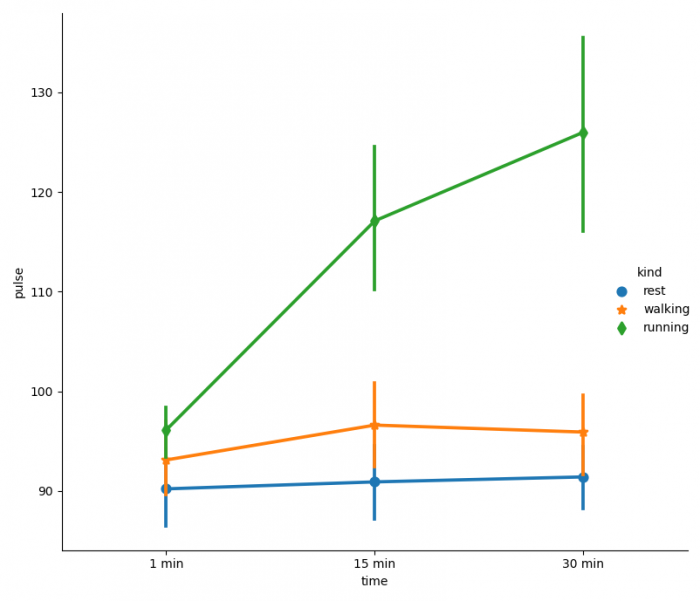# How to change the linewidth and markersize separately in a factorplot in Matplotlib?

To change the linewidth and markersize separately in a factorplot, we can use the following steps −

• Set the figure size and adjust the padding between and around the subplots.
• Load an example dataset from the online repository.
• Use factorplot() method with scale to change the marker size.
• To display the figure, use show() method.

## Example

import seaborn as sns
from matplotlib import pyplot as plt

plt.rcParams["figure.figsize"] = [7.50, 3.50]
plt.rcParams["figure.autolayout"] = True

plt.show()